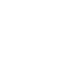# Feb 15 ACT & SAT Question of the Day

http://sat.collegeboard.org/practice/sat-question-of-the-day?src=R&questionId=20130215 (This link takes you to today’s question. If you use my archive, you will see the question related to my SAT explanation for that date.)

The answer is E.  If you took more than 5 seconds to do this question, you are about to learn a lot about the test.  Tell your friends to check this out!!

There are so many ways to do this question without doing any algebra (which I’m sure most of you did) that is is silly.  First, the SAT folks use numbers “that work,” i.e., they are easy to manipulate.  When they tell you that “3 is subtracted from x and the square root…,” you can bet the house that the difference is a perfect square!  That means the answer is most likely going to  be E.  When you subtract 3 from any of the other answers, you don’t get the normal suspect perfect squares.   The fact that answer E-3  ( 19-3) is answer D just confirms it.  Of course, once you figure out it should be 19, go ahead and do what they tell you to do in the problem just to make sure.  Start with 19 as x and see if it works.  (That’s what I did the first time through the problem.)

You may ask why did I eliminate answer B so quickly since when you subtract 3 from 4, you get 1 which is a perfect square.  Two reasons.  First, the square root of 1 is 1 and that’s a long ways from 9, adding 5 isn’t even close.  A or 3 is out since they are not going to have you take the square root of 0.  I’m not saying it is impossible for A or B to be correct but based on the SAT test writers’ habits, E is almost certainly the answer.

Another way to avoid the algebra is to start by subtracting the 5 from 9 and work backwards through the problem.  Then square 4 to get 16 and add the 3.  Bingo!  You get 19.  (That’s what I did the second time through the question but skipped doing the answers when x-3 wasn’t a perfect square.)

A third way is to start with the answers since one of them has to be x.  Whenever the question is “what is the value of…,” you know to plug in the answers and give them a try.  Start with C since it is in the middle.  Often, you’ll know which way to go with the remaining answers, up or down, if C is wrong.  That process will save you some time.  (I skipped doing it this way this morning.)

Of course, a final way to do the question is to do it the “algebra teacher way” and as is par for the course, the SAT folks kindly give you that explanation.  Good luck with finishing on time if you do all the problems on the SAT doing all the math.  If you are really extra strong at math, you’ll be able to finish on time doing all the math.  The rest of us mortals need to learn and use a few time-saving tricks like the ones above!  Enjoy the game.

http://www.act.org/qotd/ (The ACT staff does not put a date on their questions so if you click on an archived blog, you’ll get today’s question and the old explanation. Sorry. The SAT staff has dated their questions; so, the archive is helpful. The ACT folks simply don’t do that.)

The answer is A.  This is a simple “turn the math crank” problem and the ACT folks show you in their explanation how to do that

The way I did it was I realized a times the absolute value of b-2 is positive and we are multiplying.  That means a has to be negative.  That left only answers A and B.  Answer A looked good since as long as b isn’t 0, there is an absolute value other than 0.  Then I threw out answer B since it would make the absolute value zero if b=2.   All done.  That strand of thinking took less than 10 seconds.

What was the clue that got me on the right track to do the problem this way.  I saw we are multiplying and the product is negative.  There’s only one way that can happen: a positive times a negative.  I’m off and running.  The signal was <o.  Watch for it!

You could also do it another way: substitute numbers for variables, i.e., change the abstract to the concrete.  For answer A, let’s say that a is -1 and b is 3.  That works, the inequality is true.  To check your work, do the other answers.  For answer B, let’s say that a is -1 and b must be 2. That doesn’t work.  For answer C, it may or may not work depending on whether you use a positive or negative value for a.  For answer D, using any positive value for a doesn’t work.  You already know A works so you can eliminate E.

The Wizard